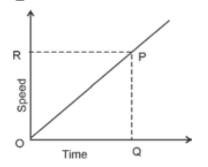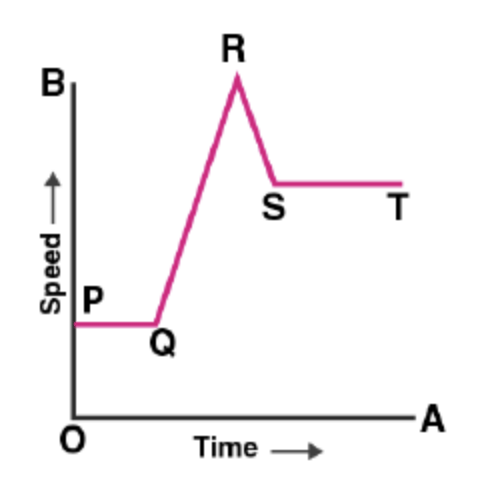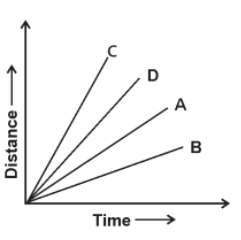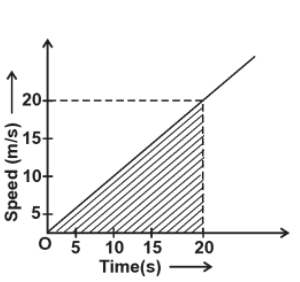Test Description

## 10 Questions MCQ Test Science Olympiad Class 9 | Olympiad Test: Motion- 1

Olympiad Test: Motion- 1 for Class 9 2023 is part of Science Olympiad Class 9 preparation. The Olympiad Test: Motion- 1 questions and answers have been prepared according to the Class 9 exam syllabus.The Olympiad Test: Motion- 1 MCQs are made for Class 9 2023 Exam. Find important definitions, questions, notes, meanings, examples, exercises, MCQs and online tests for Olympiad Test: Motion- 1 below.
 1 Crore+ students have signed up on EduRev. Have you?
Olympiad Test: Motion- 1 - Question 1

### The quantity which is measured by the area occupied under the speed-time graph is

Detailed Solution for Olympiad Test: Motion- 1 - Question 1Distance travelled = (½) × Area of rectangle OQPR

=(½) × OR × OQ

OR = speed,

OQ = time at

OP → velocity curve.

Olympiad Test: Motion- 1 - Question 2

### A bus increases its speed from 36 km/h to 72 km/h in 10 seconds. Its acceleration is

Detailed Solution for Olympiad Test: Motion- 1 - Question 2

v=72km/h=72×(5/18)=20m/s

u=36km/h=36×(5/18)=10m/s

t=10 sec.

A=(v-u)/t=(20-10)/10=1 m/s2

Olympiad Test: Motion- 1 - Question 3

### What does the slope of a velocity-time graph indicate ?

Detailed Solution for Olympiad Test: Motion- 1 - Question 3

Acceleration = change in velocity/Time taken

Olympiad Test: Motion- 1 - Question 4

An object moving with a velocity of 30 m/s decelerates at the rate of 1.5 m/s2. Find the time taken by the object to come to rest

Detailed Solution for Olympiad Test: Motion- 1 - Question 4

When a body comes to rest, v = 0, here u = 30 m/s,
a = –1.5 m/s2
v = u + at
t=(v-u)/a =(0-30)/-1.5 =20 seconds

Olympiad Test: Motion- 1 - Question 5

In the speed-time graph for a moving object shown here, the part which indicates uniform deceleration of the object isDetailed Solution for Olympiad Test: Motion- 1 - Question 5

As we move along the graph, PQ and ST show uniform acceleration. QR shows acceleration at an increasing rate. RS shows deceleration i.e.speed is decreasing.

Olympiad Test: Motion- 1 - Question 6

A train starting from rest attains a velocity of 72 km/h in 5 minutes. Assuming that the acceleration is uniform, find the distance travelled by the train for attaining this velocity

Detailed Solution for Olympiad Test: Motion- 1 - Question 6

u = 0, v = 72 km/h = 72 × (5/18)= 20 ms–1,

t = 5 minutes=300s

a =(v-u)/t =20/300=1/15 m/s2

=>v2=u2+2as=3000ssms=3kms.

Olympiad Test: Motion- 1 - Question 7

Four cyclists A, B, C, and D are cycling on a levelled straight road. Their distance–time graphs are shown in the given figure. Which of the following is correct regarding the motion of these cyclists?Detailed Solution for Olympiad Test: Motion- 1 - Question 7

Slope of the distance–time graph shows speed. The slope of line B is smallest, thus cyclist B is the slowest and cyclist C is the fastest among all four cyclists.

Olympiad Test: Motion- 1 - Question 8

A car of mass 1000 kg is moving with a velocity of 10 ms–1. If the velocity–time graph for this car is a horizontal line parallel to the time–axis, then the velocity of car at the end of 25 s will be

Detailed Solution for Olympiad Test: Motion- 1 - Question 8

A horizontal line parallel to the time–axis shows a uniform velocity throughout the motion of the object.

Olympiad Test: Motion- 1 - Question 9

What is the distance covered by a particle during the time interval of 20 seconds, for which the speed–time graph is shown in the adjacent figureDetailed Solution for Olympiad Test: Motion- 1 - Question 9

Distance = (½)× base × height=200m.

Olympiad Test: Motion- 1 - Question 10

A bus moving along a straight line at 15 m/s undergoes an acceleration 2.5 m/s2 . After 2 seconds,its speed will be,

Detailed Solution for Olympiad Test: Motion- 1 - Question 10

v = u + at

=20 m/s.